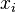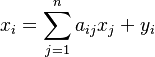# Total Output

## Definition

Total Output in the context of an Input-Output Matrix denotes the total production of a particular sector (or product). An Input-Output Model is fundamentally a system of linear equations where the Total Output of an industry is distributed through sales to other sectors and to Final Demand

## Usage

Total Output is an additional column in the IO matrix that contains the sum of Intermediate Output and final demand. It can be interpreted as market balance equation in a standard Leontief Model.

## Formula

Assume that the economy can be categorized into n sectors. Total Output is a vector, usually denoted as$x_i$, with i ranging between 1 and n which satisfies the system of linear equations:\begin{align} x_{i} & = \sum_{j=1}^{n} a_{ij} x_{j} + y_i \\ \end{align}